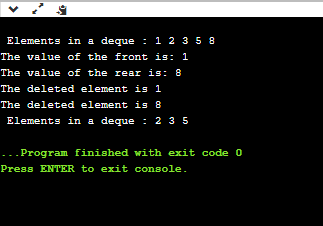# Deque

The dequeue stands for Double Ended Queue . In the queue, the insertion takes place from one end while the deletion takes place from another end. The end at which the insertion occurs is known as the rear end whereas the end at which the deletion occurs is known as front end .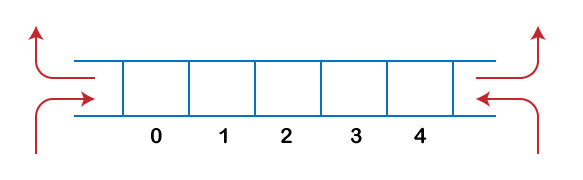Deque is a linear data structure in which the insertion and deletion operations are performed from both ends. We can say that deque is a generalized version of the queue.

Let’s look at some properties of deque.

• Deque can be used both as stack and queue as it allows the insertion and deletion operations on both ends.

In deque, the insertion and deletion operation can be performed from one side. The stack follows the LIFO rule in which both the insertion and deletion can be performed only from one end; therefore, we conclude that deque can be considered as a stack.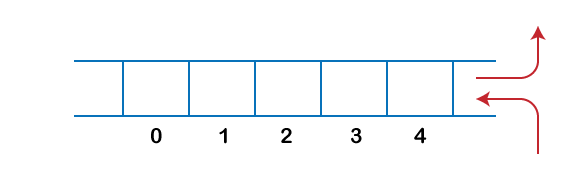In deque, the insertion can be performed on one end, and the deletion can be done on another end. The queue follows the FIFO rule in which the element is inserted on one end and deleted from another end. Therefore, we conclude that the deque can also be considered as the queue.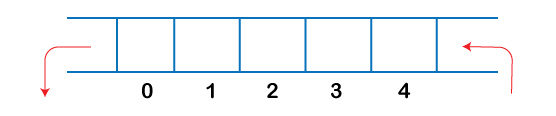There are two types of Queues, Input-restricted queue , and output-restricted queue .

1. Input-restricted queue: The input-restricted queue means that some restrictions are applied to the insertion. In input-restricted queue, the insertion is applied to one end while the deletion is applied from both the ends.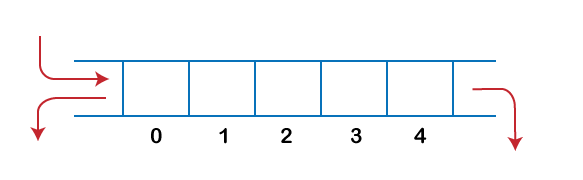2. Output-restricted queue: The output-restricted queue means that some restrictions are applied to the deletion operation. In an output-restricted queue, the deletion can be applied only from one end, whereas the insertion is possible from both ends.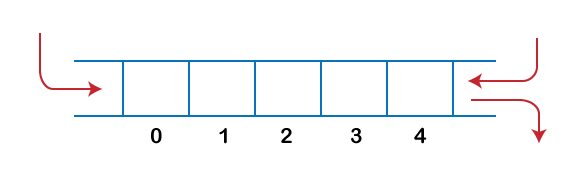### Operations on Deque

The following are the operations applied on deque:

• Insert at front
• Delete from end
• insert at rear
• delete from rear

Other than insertion and deletion, we can also perform peek operation in deque. Through peek operation, we can get the front and the rear element of the dequeue.

We can perform two more operations on dequeue:

• isFull(): This function returns a true value if the stack is full; otherwise, it returns a false value.
• isEmpty(): This function returns a true value if the stack is empty; otherwise it returns a false value.

### Memory Representation

The deque can be implemented using two data structures, i.e., circular array , and doubly linked list . To implement the deque using circular array, we first should know what is circular array .

### What is a circular array?

An array is said to be circular if the last element of the array is connected to the first element of the array. Suppose the size of the array is 4, and the array is full but the first location of the array is empty. If we want to insert the array element, it will not show any overflow condition as the last element is connected to the first element. The value which we want to insert will be added in the first location of the array.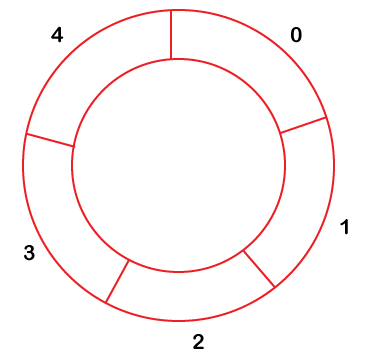### Applications of Deque

• The deque can be used as a stack and queue ; therefore, it can perform both redo and undo operations.
• It can be used as a palindrome checker means that if we read the string from both ends, then the string would be the same.
• It can be used for multiprocessor scheduling. Suppose we have two processors, and each processor has one process to execute. Each processor is assigned with a process or a job, and each process contains multiple threads. Each processor maintains a deque that contains threads that are ready to execute. The processor executes a process, and if a process creates a child process then that process will be inserted at the front of the deque of the parent process. Suppose the processor P2 has completed the execution of all its threads then it steals the thread from the rear end of the processor P1 and adds to the front end of the processor P2. The processor P2 will take the thread from the front end; therefore, the deletion takes from both the ends, i.e., front and rear end. This is known as the A-steal algorithm for scheduling.

### Implementation of Deque using a circular array

The following are the steps to perform the operations on the Deque:

### Enqueue operation

1. Initially, we are considering that the deque is empty, so both front and rear are set to -1, i.e., f = -1 and r = -1 .
2. As the deque is empty, so inserting an element either from the front or rear end would be the same thing. Suppose we have inserted element 1, then front is equal to 0, and the rear is also equal to 0.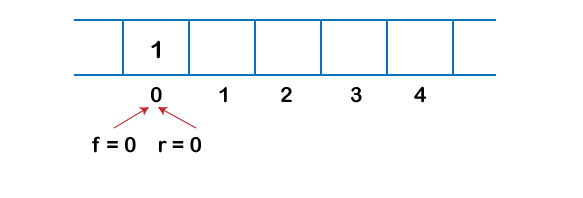3. Suppose we want to insert the next element from the rear. To insert the element from the rear end, we first need to increment the rear, i.e., rear=rear+1 . Now, the rear is pointing to the second element, and the front is pointing to the first element.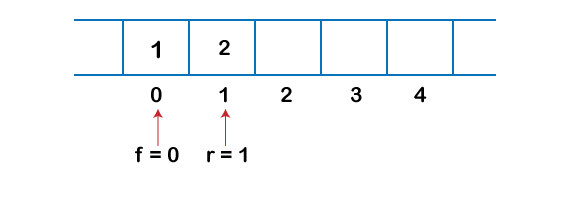4. Suppose we are again inserting the element from the rear end. To insert the element, we will first increment the rear, and now rear points to the third element.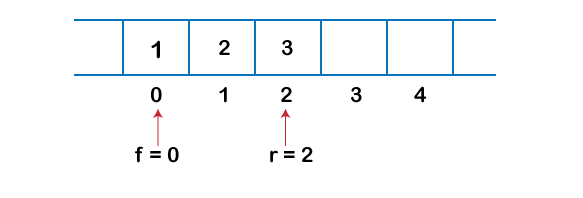5. If we want to insert the element from the front end, and insert an element from the front, we have to decrement the value of front by 1. If we decrement the front by 1, then the front points to -1 location, which is not any valid location in an array. So, we set the front as (n -1), which is equal to 4 as n is 5. Once the front is set, we will insert the value as shown in the below figure: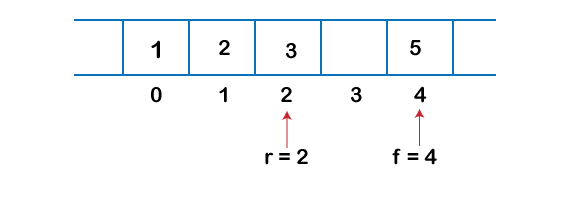### Dequeue Operation

1. If the front is pointing to the last element of the array, and we want to perform the delete operation from the front. To delete any element from the front, we need to set front=front+1 . Currently, the value of the front is equal to 4, and if we increment the value of front, it becomes 5 which is not a valid index. Therefore, we conclude that if front points to the last element, then front is set to 0 in case of delete operation.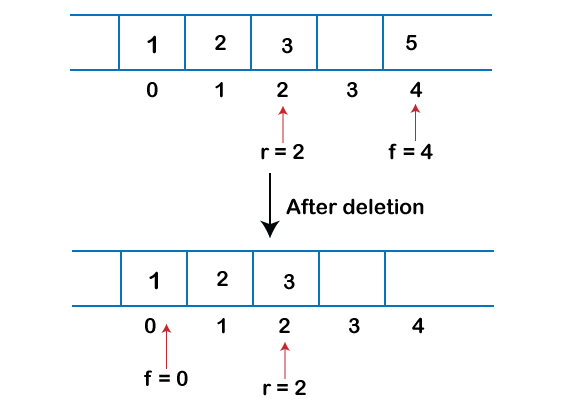2. If we want to delete the element from rear end then we need to decrement the rear value by 1, i.e., rear=rear-1 as shown in the below figure: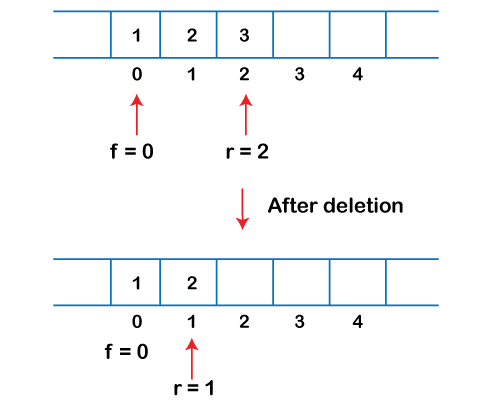3. If the rear is pointing to the first element, and we want to delete the element from the rear end then we have to set rear=n-1 where n is the size of the array as shown in the below figure: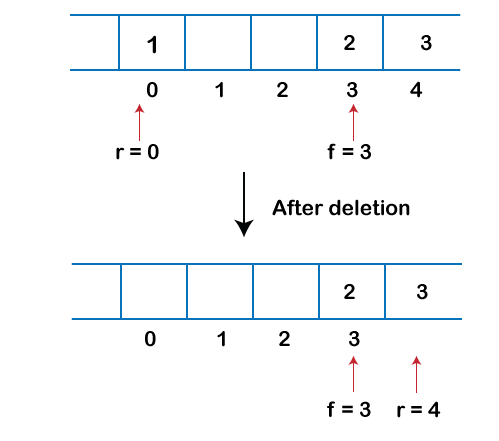Let’s create a program of deque.

The following are the six functions that we have used in the below program:

• enqueue_front(): It is used to insert the element from the front end.
• enqueue_rear(): It is used to insert the element from the rear end.
• dequeue_front(): It is used to delete the element from the front end.
• dequeue_rear(): It is used to delete the element from the rear end.
• getfront(): It is used to return the front element of the deque.
• getrear(): It is used to return the rear element of the deque.
``````#define size 5
#include <stdio.h>
int deque[size];
int f=-1, r=-1;
//  enqueue_front function will insert the value from the front
void enqueue_front(int x)
{
if((f==0 && r==size-1) || (f==r+1))
{
printf("deque is full");
}
else if((f==-1) && (r==-1))
{
f=r=0;
deque[f]=x;
}
else if(f==0)
{
f=size-1;
deque[f]=x;
}
else
{
f=f-1;
deque[f]=x;
}
}

// enqueue_rear function will insert the value from the rear
void enqueue_rear(int x)
{
if((f==0 && r==size-1) || (f==r+1))
{
printf("deque is full");
}
else if((f==-1) && (r==-1))
{
r=0;
deque[r]=x;
}
else if(r==size-1)
{
r=0;
deque[r]=x;
}
else
{
r++;
deque[r]=x;
}

}

// display function prints all the value of deque.
void display()
{
int i=f;
printf("\n Elements in a deque : ");

while(i!=r)
{
printf("%d ",deque[i]);
i=(i+1)%size;
}
printf("%d",deque[r]);
}

// getfront function retrieves the first value of the deque.
void getfront()
{
if((f==-1) && (r==-1))
{
printf("Deque is empty");
}
else
{
printf("\nThe value of the front is: %d", deque[f]);
}

}

// getrear function retrieves the last value of the deque.
void getrear()
{
if((f==-1) && (r==-1))
{
printf("Deque is empty");
}
else
{
printf("\nThe value of the rear is: %d", deque[r]);
}

}

// dequeue_front() function deletes the element from the front
void dequeue_front()
{
if((f==-1) && (r==-1))
{
printf("Deque is empty");
}
else if(f==r)
{
printf("\nThe deleted element is %d", deque[f]);
f=-1;
r=-1;

}
else if(f==(size-1))
{
printf("\nThe deleted element is %d", deque[f]);
f=0;
}
else
{
printf("\nThe deleted element is %d", deque[f]);
f=f+1;
}
}

// dequeue_rear() function deletes the element from the rear
void dequeue_rear()
{
if((f==-1) && (r==-1))
{
printf("Deque is empty");
}
else if(f==r)
{
printf("\nThe deleted element is %d", deque[r]);
f=-1;
r=-1;

}
else if(r==0)
{
printf("\nThe deleted element is %d", deque[r]);
r=size-1;
}
else
{
printf("\nThe deleted element is %d", deque[r]);
r=r-1;
}
}

int main()
{
// inserting a value from the front.
enqueue_front(2);
// inserting a value from the front.
enqueue_front(1);
// inserting a value from the rear.
enqueue_rear(3);
// inserting a value from the rear.
enqueue_rear(5);
// inserting a value from the rear.
enqueue_rear(8);
// Calling the display function to retrieve the values of deque
display();
// Retrieve the front value
getfront();
// Retrieve the rear value.
getrear();
// deleting a value from the front
dequeue_front();
//deleting a value from the rear
dequeue_rear();
// Calling the display function to retrieve the values of deque
display();
return 0;
}
``````

Output: